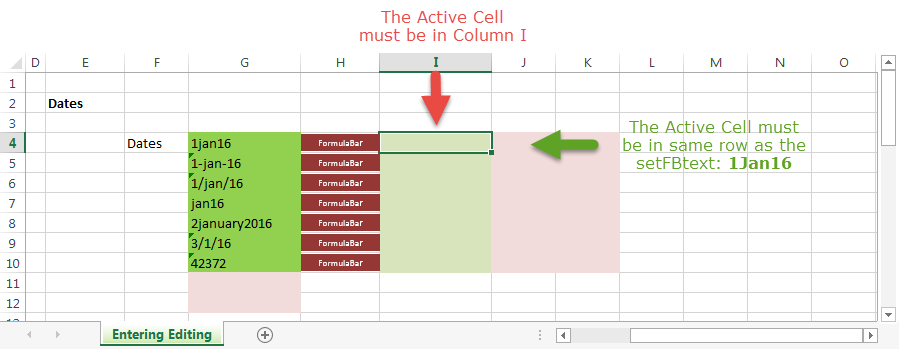setFormulaBar text

xlf | setFormulaBarText

Send selected text to the Formula Bar in edit mode

Speed up workshop sessions by sending selected text to the Formula Bar. This saves excessive typing of data by participants in session workshops. The example data in figure 1 is based on the entering and editing worksheet data module.

To use the setFormulaBarText macro

• The source text is in column G. The setFormulaBarText macro will only accept source data from cells with a Light Green fill colour.
• To send the text in cell G4 (the cell to the left of the FormulaBar button) to the Formula Bar
• Select the target cell in column I (the cell to the right of the FormulaBar button) on the same row
• Click the FormulaBar button. 1Jan16 will appear in the Formula bar
• Complete the entry by pressing the Enter keyFig 1: setFormulaBarText worksheet example - the Active Cell must be in Column I, and the source text 2 cells to the left. Column G - same row

If the user selects an incorrect column, or a row without source data of the correct format, then an error message is displayed - see figure 2.

setFormulaBarText - the VBA code

The macro is based on the Application.SendKeys method. The SendKeys method sends keystrokes as text to the active application.

Code 1: Sub procedure setFormulaBarText add xlf blue fill colour to selected area of worksheet
Private Sub setFormulaBarText()
Dim tmp As String
Dim MBprompt As String, MBtitle As String
Dim ACColumn As Long        '' ActiveCell column
Dim ACOffsetColor As Double

MBprompt = "For correct operation ensure that: " & vbNewLine & vbNewLine & _
"1. The ActiveCell is in Column I, and" & vbNewLine & _
"2. The ActiveCell is in the same row as the Formula Input Cell in Column G" & vbNewLine & _
"3. The Formula Input cell has Standard Fill Color: Light Green"
MBtitle = "xlf | setFormulaBarText macro button ERROR"

If Application.Version = "12.0" Or "14.0" Or "15.0" Then
ACColumn = ActiveCell.Column
ACOffsetColor = ActiveCell.Offset(0, -2).Interior.Color
If Not (ACColumn = 9 And ACOffsetColor = 5296274) Then
MsgBox MBprompt, , MBtitle
Exit Sub
End If
'' Replace Shift +, Control ^ and Alt % key codes
tmp = ActiveCell.Offset(0, -2).Value
tmp = Replace(tmp, "+", "{+}")
tmp = Replace(tmp, "^", "{^}")
tmp = Replace(tmp, "%", "{%}")
ActiveCell.ClearContents
Application.SendKeys "{F2}" & tmp
Else
MsgBox "Procedure requires Excel 2007 or later"
End If

End Sub


The FormulaBar button

• The FormulaBar button is a Rectangle object from the ribbon Insert > Illustrations > Shapes > Rectangle sequence
• Solid Fill, Theme Color: Red, Accent 2, Darker 25%
• Properties: Move and size with cells
• Font: 7 point
• Assign Macro: 'xlf-session-1.xlsm'!setFormulaBarText

• This example was developed in Excel 2013 Pro 64 bit.
• Revised: Tuesday 8th of December 2015 - 07:03 PM, [Australian Eastern Standard Time (EST)]